Biomedical Statistics and Informatics
Volume 1, Issue 1, December 2016, Pages: 13-18

A Model Selection on Economic Variable in Nigeria

Muritala Abdulkabir1, Omuku Ikechukwu Joshua1, Raji Surajudeen Tunde2

1Statistics Department, Lens Polytechnic Offa, Offa, Nigeria

2Mathematics and Statistics Department, Federal Polytechnic Offa, Offa, Nigeria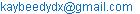(M. Abdulkabir)

Muritala Abdulkabir, Omuku Ikechukwu Joshua, Raji Surajudeen Tunde. A Model Selection on Economic Variable in Nigeria. Biomedical Statistics and Informatics. Vol. 1, No. 1, 2016, pp. 13-18.doi: 10.11648/j.bsi.20160101.12

Received: September 11, 2016; Accepted: October 21, 2016; Published: December 12, 2016

Abstract: This study is on model selection on economic variable on gross domestic product in Nigeria, the data used for this study were extracted from National Bureau of Statistics (NBS), the statistical tool is multiple regression model and model selection to select the best model and in the variable and to evaluate and test GDP as a determinant which will capture the effect on economic variables. At the end of the analysis and findings it were concluded that Import value import value from the export, production, petroleum and consumption plays the most significant role in the company’s market. It can be used as a tool to estimate the company’s future market price.

Keywords: Gross Domestics Product, Multiple Regression, Model Selection, Variance Inflation Factor (VIF), Tolerance

Contents

1. Introduction

The responsibility shouldered by the government of any nation, particularly the developing nations, is enormous. The need to fulfil these responsibilities largely depends on the amount of revenue generated by the government through various means. Taxation is one of the oldest means by which the cost of providing essential services for the generality of persons living in a given geographical area is funded. Globally, governments are saddled with the responsibility of providing some basic infrastructures for their citizens. Functions or obligations the government may owe her citizens include but are not restricted to: stabilization of the economy, redistribution of income and provision of services in the form of public goods .

Taxation is a major source of government revenue all over the world and governments use tax proceeds to render their traditional functions, such as: the provision of goods, maintenance of law and order, defence against external aggression, regulation of trade and business to ensure social and economic maintenance . The primary function of a tax system is to raise enough revenue to finance essential expenditures on the goods and services provided by government; and tax remains one of the best instruments to boost the potential for public sector performance and repayment of public debt . A system of tax avails itself as a veritable tool that mobilizes a nation’s internal resources and it lends itself to creating an environment that is conducive for the promotion of economic growth . Therefore, taxation plays a major role in assisting a country to meet its needs and promote self-reliance.

In Nigeria, tax revenue has accounted for a small proportion of total government revenue over the years compared with the bulk of revenue needed for development purposes that is derived from oil . The serious decline in the prices of oil in recent times has led to a decrease in the funds available for distribution to the federal, state and local governments . Consequently, dependence on oil as a particular or main source of revenue in Nigeria has become risky and not beneficial for sustainable economic growth. It is worse for Nigeria where there are fluctuations in prices in the oil market; thereby creating concerns amongst Nigerians and indeed the Nigerian government on the need to diversify the economy.

Naturally, and globally, there is a paradigm shift to taxation revenue as an alternative source of revenue. Nigeria is not an exception. The machinery and procedures for implementing a good tax system in Nigeria are inadequate; hence tax evasion and avoidance of the self-employed individuals and organizations whose data base is not captured in the relevant tax authority’s data system . The need for the government to generate adequate revenue from internal sources has therefore become a matter of extreme urgency and importance . The desire of any government to maximize revenue from taxes collected from tax payers cannot be overemphasized.

This is because, as it well-known, the importance of tax lies in its ability to generate revenue for the government, influence the consumption trends and grow and regulate economy through its influence on vital aggregate economic variables . In the light of the above, and in broad spectrum, this paper examines the impact of indirect taxes on economic growth in Nigeria. This topic is formed and informed against the backdrop of the need for a paradigm shift to indirect taxation in the face of the dwindling oil prices and the relative paucity of studies, using inflation-factored GDP in Nigeria. To this end, and in order to set a direction for

This paper, aim to evaluate and test GDP as a determinant which will capture the effect on economic variables and identify the best model in the fitted model using the model selection approach

2. Methodology

Regression analysis:

Regression analysis is a technique used in statistics for investigating and modeling the relationship between variables .

Simple linear regression:

Simple linear regression is a model with a single regressor x that has a relationship with a response y that is a straight line. This simple linear regression model can be expressed as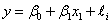where the intercept β0 and the slope β1 are unknown constants and ε is a random error component.

Multiple linear regression:

If there is more than one regressor, it is called multiple linear regression. In general, the response variable y may be related to k regressors, x1, x2,…,xk, so that

y = β0 + β1x1 + β2x2 +…+ βkxk + ε

Least Squares Estimation:

The method of least squaresis used to estimate β0, β1,… βk. That is, we estimate β0 and β1 so that the sum of the squares of the differences between the observations yi and the straight line is a minimum .

R-squared:

R-squaredis a measure in statistics of how close the data are to the fitted regression line. It is also known as the coefficient of determination, or the coefficient of multiple determinations for multiple regression. It is the percentage of the response variable variation that is explained by a linear model.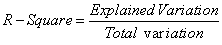R-squared is always between 0 and 100%. 0% means the model explains none of the variability of the response data around its mean. 100% indicates that the model explains all the variability of the response data around its mean. Generally, the higher the R-squared, the better the model fits the data (Frost, 2013).

Analysis of variance (ANOVA):

Analysis of variance (ANOVA) is a collection of statistical models used in order to analyze the differences between group means and their associated procedures. In the ANOVA setting, the observed variance in a particular variable is partitioned into components attributable to different sources of variation. The following equation is the Fundamental Analysis-of-Variance Identity for a regression model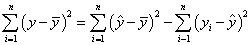SST = SSR + SSRes

Statistical Hypothesis:

Statistical hypothesisare statements about relationships. The statistical hypothesis testing is the use of statistics to determine the probability that a given hypothesis is true . The null hypothesis is denoted by

H0. The alternative hypothesis is the negation of the null hypothesis, denoted by

H1 or Ha

Testing Significance of Regression:

H0: β1 = β2 =…= βk = 0

H1: at least one βi ≠ 0

The hypotheses are related to the significance of regression. Failing to reject H0 implies that there is no linear relationship between x and y. On the other hand, if H0 is rejected, it implies that at least one βi show a significant relationship to y

F-test:

An F-testis a statistical test in which the test statistic is based on the F-distribution under the null hypothesis. It is most often used when comparing statistical models that have been fitted to a data set, in order to identify the model that best fits the population from which the data were sampled. In this research, the F-test is used to test the significance of the model.

The test statistics F0 can be computed by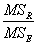follows the Fk,n-k-1 distribution. Reject H0, if F0> Fk,n-k-1. The test statistic F0 can usually be obtained from the ANOVA table

Test on Individual Regression Coefficients (t Test):

The t-testis used to check the significance of individual regression coefficients in the multiple linear regression model. Adding a significant variable to a regression model makes the model more effective, while adding an unimportant variable may make the model worse. The hypothesis statements to test the significance of a particular regression coefficient, βj, are:

H0: βj = 0

H1: βj ≠ 0

The test statistic for this test has the t-distribution: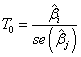where the standard error, s e(β j), is obtained. One would fail to reject the null hypothesis if the test statistic lies in the acceptance region: -tα/2, n-2< T0< tα/2, n-2

This test measures the contribution of a variable while some other variables are included in the model

P-value:

P-value or calculated probability is the estimated probability of rejecting the null hypothesis (H0) of a study question when that hypothesis is true.

The variance Inflation Factor (VIF):

The variance inflation factor for each term in the model measures the combined effect of the dependences among the regressors on the variance of the term. Practical experience indicates that if any of the VIFs exceeds 5 or 10, it is an indication that the associated regression coefficients are poorly estimated because of multicollinearity

Model Selection:

1.) Forward Selection: This procedure begins with the assumption that there is no regressor in the model other than the intercept. The goal is to find an optimal subset by inserting regressors into the model one at a time.

2.) Backward Elimination: This procedure is the opposite approach from the forward selection. First, we begin with a full model with K candidate regressors. Then, the partial F statistic (or a t statistic) is computed for each regressor. If the regressor with the smallest partial F or t value is less than the preselected F value, that regressor is removed from the model. Fit model with K-1 predictors and the procedure is repeated.

3.) Stepwise Regression: It is a method that allows moves in either direction, dropping or adding variables at the various steps. It combines both forward selection and backward elimination. We perform two steps in forward selection and a backward step. Then, perform another forward step and another backward step. We continue until no action can be taken in either direction

Residuals:

The difference between the observed value of the dependent variable (y) and the predicted value (ŷ) is called theresidual(e). Each data point has one residual.

Residual = Observed value - Predicted value

e = y - ŷ

Both the sum and the mean of the residuals are equal to zero. That is,

Σ e = 0 and e = 0 .

Residual Diagnostics:

A residual plotis a graph that shows the residuals on the vertical axis and the independent variable on the horizontal axis. If the points in a residual plot are randomly dispersed around the horizontal axis, a linear regression model is appropriate for the data; otherwise, a non-linear model is more appropriate.

Checking normality:

Histogram:

The Histogram of the Residual can be used to check whether the variance is normally distributed. A symmetric histogram as shown in the figure 1 below, which is evenly distributed around zero, indicates that the normality assumption is likely to be true . The typical"bell-curve" is the ideal indication as to normality. When this cannot be obtained, a symmetrical histogram is sufficient.

3. Analysis

Table 1. Descriptive statistics.

 Descriptive Statistics Mean Std. Deviation N Market price 224200.1333 184297.60461 15 Export 57251.4667 29926.93610 15 Petroleum 51906.6667 28290.41561 15 Import 33395.9333 20508.49817 15 Production 2030.1600 189.77164 15 Consumption 269.0867 60.73901 15

The descriptive statistics in table 1 shows the summary of market price, export, petroleum, import, production and consumption with average and standard deviation with sample size of 15

Table 2. Model coefficient Ordinary Least Square.

 Coefficientsa Model Unstandardized Coefficients Standardized Coefficients t Sig. B Std. Error Beta 1 (Constant) -215284.381 149631.884 -1.439 .184 Export 6.145 2.591 .998 2.372 .042 Petroleum -5.890 2.966 -.904 -1.986 .078 Import 4.428 .994 .493 4.454 .002 Production -61.378 51.157 -.063 -1.200 .261 Consumption 1375.489 283.863 .453 4.846 .001

a. Dependent Variable: Market price

Market Price=-215284.381+6.145Export-5.890Petroleum+4.428Import-61.378+1375.489consumption

Test of Hypothesis

H0: β1 = 0 (The linear model is inadequate).

H1: β1 ≠ 0 (The linear model is adequate).

Level of significance α=0.05

Computation

Table 3. Model summary.

 Model Summary Model R R Square Adjusted R Square Std. Error of the Estimate 1 .994a .987 .980 26149.66007

a. Predictors: (Constant), Consumption, Production, Export, Import, Petroleum

Table 4. Analysis of Variance (ANOVA).

 ANOVAa Model Sum of Squares df Mean Square F Sig. 1 Regression 469364256419.686 5 93872851283.937 137.280 .000b Residual 6154242494.047 9 683804721.561 Total 475518498913.733 14

a. Dependent Variable: Market price

b. Predictors: (Constant), Consumption, Production, Export, Import, Petroleum

Decision rule: Reject Ho if P-value is less than level of significant α=0.05 otherwise do not reject Ho

Decision: Ho is rejected

Conclusion: It can be concluded the model is adequate

4. Model Selection

Forward Selection Method

Table 5. Model coefficient.

 Coefficientsa Model Unstandardized Coefficients Standardized Coefficients t Sig. B Std. Error Beta 1 (Constant) -63778.521 27238.050 -2.342 .036 Import 8.623 .701 .960 12.293 .000 2 (Constant) -276539.812 52673.251 -5.250 .000 Import 5.939 .775 .661 7.659 .000 Consumption 1123.797 261.839 .370 4.292 .001

a. Dependent Variable: Market price

The final regression equation from the above model which indicate the best model from the Variance Inflation Factor is Market price=-63778.521+8.623Import

Backward Elimination:

The market price is perfectly explained by all of the variables combined, so the standard error is zero. The test of statistics is undefined when regressing market price with the five (5) main factors on linear regression. In order to further use Backward Elimination in pinpointing a factor’s contribution to the market price of a company, every variable, but one (K-1) are selected to generate the initial model for this method.

Table 6. Model Coefficient.

 Coefficientsa Model Unstandardized Coefficients Standardized Coefficients t Sig. B Std. Error Beta 1 (Constant) -215284.381 149631.884 -1.439 .184 Export 6.145 2.591 .998 2.372 .042 Petroleum -5.890 2.966 -.904 -1.986 .078 Import 4.428 .994 .493 4.454 .002 Production -61.378 51.157 -.063 -1.200 .261 Consumption 1375.489 283.863 .453 4.846 .001 2 (Constant) -384224.238 51731.458 -7.427 .000 Export 7.565 2.354 1.228 3.213 .009 Petroleum -7.702 2.608 -1.182 -2.953 .014 Import 4.626 1.002 .515 4.618 .001 Consumption 1563.062 242.084 .515 6.457 .000

a. Dependent Variable: Market price

The model for the backward selection is

y=-215284.381+6.145Export-5.890Petroleum-61.378Production+1375.48Consumption

Table 7. Stepwise Selection.

 Coefficientsa Model Unstandardized Coefficients Standardized Coefficients t Sig. B Std. Error Beta 1 (Constant) -63778.521 27238.050 -2.342 .036 Import 8.623 .701 .960 12.293 .000 2 (Constant) -276539.812 52673.251 -5.250 .000 Import 5.939 .775 .661 7.659 .000 Consumption 1123.797 261.839 .370 4.292 .001

a. Dependent Variable: Market price

The model equation from the above model which indicate the best model from the Variance Inflation Factor is Market price=-63778.521+8.623 Import which is the same as the forward selection

Findings of the best model selection using simple linear regression (i.e Market price vs Import)

Table 8. Model Coefficient.

 Coefficientsa Model Unstandardized Coefficients Standardized Coefficients t Sig. B Std. Error Beta 1 (Constant) -63778.521 27238.050 -2.342 .036 Import 8.623 .701 .960 12.293 .000

a. Dependent Variable: Market price

The model is Market price=-63778.521+8.623Import

Table 9. Test of Hypothesis.

 ANOVAa Model Sum of Squares df Mean Square F Sig. 1 Regression 437853853802.784 1 437853853802.784 151.126 .000b Residual 37664645110.950 13 2897280393.150 Total 475518498913.733 14

a. Dependent Variable: Market price

b. Predictors: (Constant), Import

H0: β1 = 0 (The relationship between Market price and value of Import is not significant).

H1: β1 ≠ 0 (The relationship between Market price and value of Import is significant).

Level of significance α=0.05

Computation

Decision rule: Reject Ho if P-value is less than level of significant α=0.05 otherwise do not reject Ho

Decision: Ho is rejected

Conclusion: from the result of the analysis it can be concluded that there is relationship between Market priceandvalue of Import is significant

Therefore the equation that best depict the market price is

Market price=-63778.521+8.623Import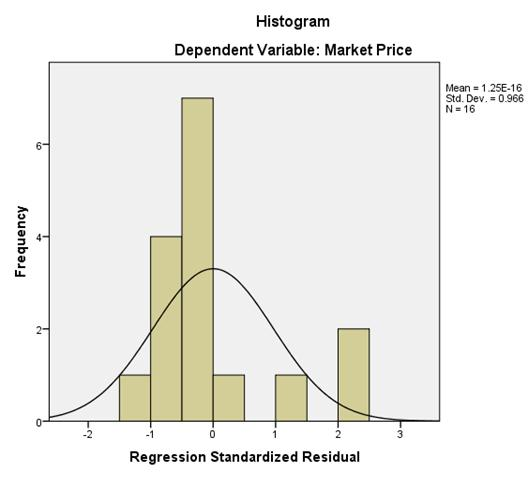Fig. 1. Normality plot.

The histogram (as shown in figure 1) appears to be symmetric, which seems to depict normality.

5. Conclusions

Based on the result of the analysis, model selection method is processed; the consensus has shown that only the Import plays a statistically significant role in the market of the company.

After the significant category is found deeper analysis is conducted of the import category. Each individual of the independent (i.e. export, petroleum, import, production and consumption) is then regressed on the market price. After running these additional models, it was discovered that the import value from the export, production, petroleum and consumption remained the most significant model.

Futhermore, it can be concluded that Import value import value from the export, production, petroleum and consumption plays the most significant role in the company’s market. It can be used as a tool to estimate the company’s future market price.

References

1. Abiola, J., & Asiweh, M. (2012). Impact of tax administration on government revenue in a developing economy- a case study of Nigeria.International Journal of Business and Social Science. 3(8), 99-113.
2. Afuberoh, D., & Okoye, E. (2014). The impact of taxation on revenue generation in Nigeria. A study of Federal Capital Territory and selected states. International Journal of Public Administration and Management Research. 2(2), 22-47.
3. Ayuba, A. J. (2014). Impact of non-oil revenue on economic growth: the Nigerian perspective. International Journal of Finance and Accounting. 3(5), 303-309.Berman, H. (n.d.). Residual Analysis in Regression. Stat Trek. Retrieved from http://stattrek.com/regression/residual-analysis.aspx
4. Douglas Montgomery, Peck, E., &Vinning, G. (2012). Introduction to LinearRegression Analysis(5th ed.).Wiley. Experiment Design and Analysis Reference. (n.d.). ReliaSoft. Retrieved from http://reliawiki.org/index.php/Experiment_Design_and_Analysis_Reference
5. Iyanaga, S., & Kawada, Y. (1980). Statistical Estimation and Statistical Hypothesis Testing. (Vol. Appendix A, Table 23). Cambridge, MA: MIT Press.
6. Otu, O. H., &Adejumo, T. O. (2013). The effects of tax revenue on economic growth in Nigeria (1970-2011). International Journal of Humanitiesand Social Science invention. 2(6), 16-26.
7. Edame, G. E., & Okoi, W. W. (2014). The impact of taxation on investment and economic development in Nigeria. Academic Journal ofInterdisciplinary Studies. 3(4). 209-218.
8. Fasoranti, M. M. (2013). Tax productivity and economic growth. Lorem Journal of Business and Economics. 1(1), 1-10.
9. Okoye, P. V. C., &Ezejiofor, R. (2014). The impact of e-taxation on revenue generation in Enugu, Nigeria. International of Advanced Research. 2(2), 449-458.

 Contents 1. 2. 3. 4. 5.
Article ToolsAbstractPDF(231K)